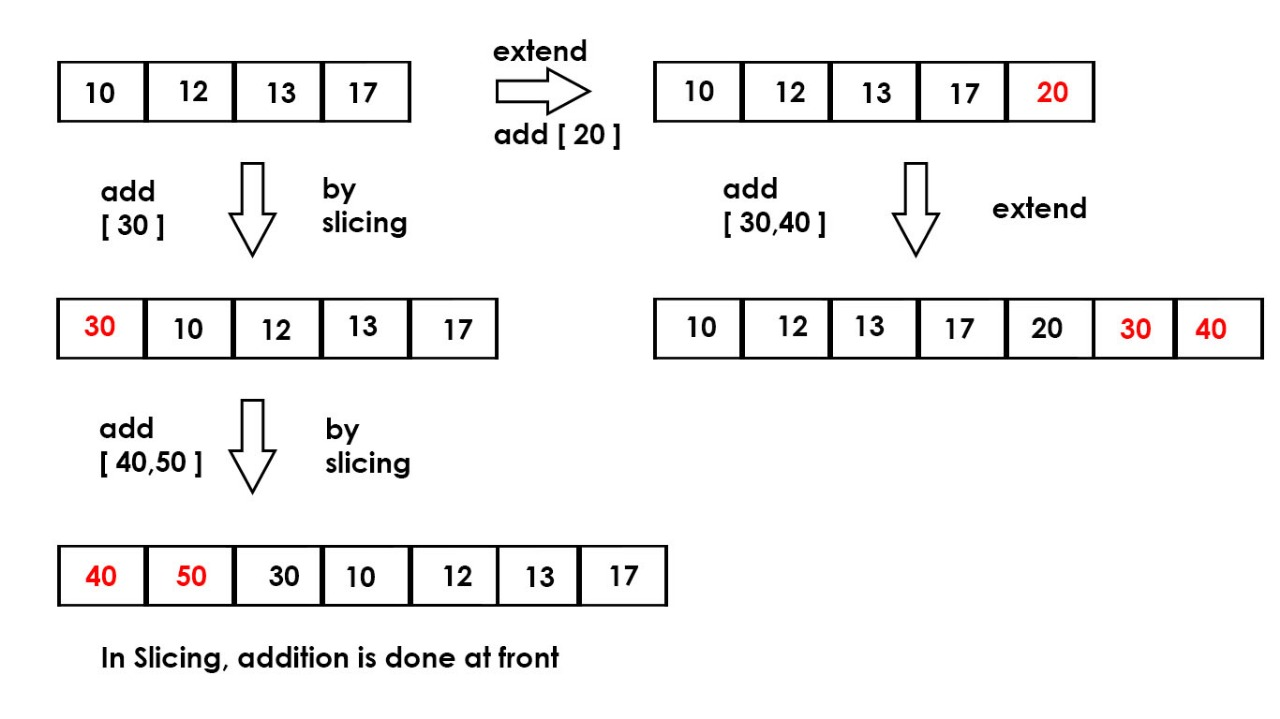# Extending a list in Python (5 different ways)

Extending a list in python can be done is following ways:
1. Using append() function: We can append at the end of the list by using append() function. For appending any single value to the list or appending a list to the list, the syntax stays the same. But we can only append a single value at a time using append() function

 `# Python program to extend a list using append() ` ` `  `a ``=` `[``10``, ``12``, ``13``, ``17``]  ` ` `  `# appending multiple values ` `a.append(``20``) ` `a.append(``22``) ` `print``(a) `

Output:

```[10, 12, 13, 17, 20, 22]
```

2. Using ‘+’ operator: We can add values by using the “+” operator. We can use [] to add any number of values to the list. Adding multiple values can be done by using ‘, ‘ values.

 `# Python program to extend a list using '+'  ` ` `  `a ``=` `[``10``, ``12``, ``13``, ``17``]  ` ` `  `# Appending single value ` `a ``=` `a ``+` `[``20``] ` ` `  `# append more then one values ` `a ``=` `a ``+` `[``30``, ``40``] ` `print``(a) `

Output:

```[10, 12, 13, 17, 20, 30, 40]
```

3. Using slicing: Using slicing in python, single or multiple values can be added to a list.

a[:0] = [x, y, z…]

Here a is the list in which the values(x, y, z..) are to be added. In this method the values are appended to the front of the list.`# Python program to extend a list using 'slicing'  ` ` `  `# appending multiple value  ` `a ``=``[``10``, ``12``, ``13``, ``17``]  ` ` `  `# add 1 number ` `a[:``0``] ``=` `[``30``] ` ` `  `# add two numbers ` `a[:``0``] ``=` `[``40``, ``50``] ` `print``(a) `

Output:

```[40, 50, 30, 10, 12, 13, 17]
```

4.Using chain(): Using chain() iterator function, we can extend a list by the syntax:

list(chain(a, [x, y, z..]))

Here a is the list in which the values(x, y, z..) are to be added. In this method the values are appended to the end of the list.

 `# python program to extend a list using  ` `# "chain" iterator functions ` `from` `itertools ``import` `*` ` `  `a ``=` `[``10``, ``20``, ``30``] ` ` `  `# extend a list ` `print``(``list``(chain(a, [``40``, ``50``, ``60``]))) `

Output:

```[10, 20, 30, 40, 50, 60]
```

5. Using Extend

 `# Python program to extend a list using extend()  ` `a ``=` `[``10``, ``12``, ``13``, ``17``]  ` ` `  `b ``=` `[``30``, ``40``] ` ` `  `a.extend(b) ` ` `  `print``(a) `

Output:

```[10, 12, 13, 17, 30, 40]
```

Attention geek! Strengthen your foundations with the Python Programming Foundation Course and learn the basics.

To begin with, your interview preparations Enhance your Data Structures concepts with the Python DS Course.

My Personal Notes arrow_drop_upStriver(underscore)79 at Codechef and codeforces D

If you like GeeksforGeeks and would like to contribute, you can also write an article using contribute.geeksforgeeks.org or mail your article to contribute@geeksforgeeks.org. See your article appearing on the GeeksforGeeks main page and help other Geeks.

Please Improve this article if you find anything incorrect by clicking on the "Improve Article" button below.

Improved By : Akanksha_Rai

Article Tags :
Practice Tags :

Be the First to upvote.

Please write to us at contribute@geeksforgeeks.org to report any issue with the above content.